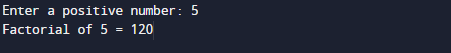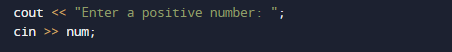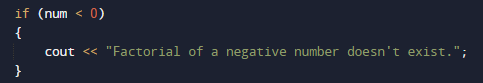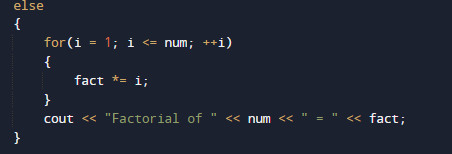# C++ Program to Find Factorial

In this tutorial you will learn about the C++ Program to Find Factorial and its application with practical example.

In this tutorial, we will learn to create a C++ program that  will Find Factorial of a number  using C++ programming.

## Prerequisites

Before starting with this tutorial we assume that you are best aware of the following C++ programming topics:

• Operators.
• Basic input/output.
• Basic c++ programming
• If-else Statement.

## What is Factorial ?

Factorial of a number n is the product of all the positive integers numbers.

Example: The factorial of 6 is 120.
6! = 6* 5 * 4 * 3 * 2 *1
6! = 720.

Factorial of 0 and 1 is 1.and  factorial of negative number doesn’t exist.

## C++ Program to Find Factorial

In this program we will find factorial of a given number . We would first declared and initialized the required variables. Next, we would prompt user to input a number .Later we will Find Factorial.

## OutputIn the above program, we have first declared and initialized a set variables required in the program.

• num = it will hold entered number.
• fact = it will hold a factorial of a number.
• i =  for iteration.

In our program, we will find factorial of a given number.
First of all we take a number as input from user and store the value in variable num.And now we will check first that the given number is greater than 1 or not if not we show message in below.And after that in else we will fin the factorial of a given number.In our program above, the user is promoted to enter a positive integer.In the our program, the loop runs from 1 to num. In each iteration , fact is multiplied with i. fact=fact*i and final value of fact is the product of all numbers between 1 to num.In this tutorial we have learn about the C++ Program to Find Factorial and its application with practical example. I hope you will like this tutorial.